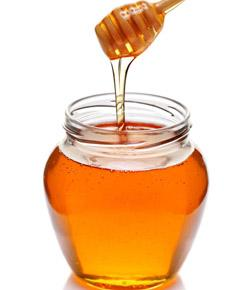# Fluids, Viscostiy, & Density

Approved & Edited by ProProfs Editorial Team
The editorial team at ProProfs Quizzes consists of a select group of subject experts, trivia writers, and quiz masters who have authored over 10,000 quizzes taken by more than 100 million users. This team includes our in-house seasoned quiz moderators and subject matter experts. Our editorial experts, spread across the world, are rigorously trained using our comprehensive guidelines to ensure that you receive the highest quality quizzes.
| By Dlindsay
D
Dlindsay
Community Contributor
Quizzes Created: 1 | Total Attempts: 635
Questions: 9 | Attempts: 644SettingsThis quiz is comprised of three  topics: Fluid, Viscosity, & Density.

• 1.

### What are fluids?

• A.

Substances that flow

• B.

Liquids, and gases

• C.

A&b

• D.

Non of the above

C. A&b
Explanation
Fluids are substances that can flow and take the shape of their container. Liquids and gases are both examples of fluids. Liquids have a definite volume but no definite shape, while gases have neither a definite volume nor shape. Therefore, the correct answer is a&b, as both liquids and gases are examples of fluids.

Rate this question:

• 2.

### Liquids & _____ are regarded as fluids.

gases
Explanation
Liquids and gases are both considered fluids because they both have the ability to flow and take the shape of their container. Unlike solids, which have a fixed shape and volume, fluids are able to move and change their shape easily. Liquids have a definite volume but no fixed shape, while gases have neither a fixed shape nor volume. Therefore, the correct answer is gases.

Rate this question:

• 3.

• 4.

### The ..................... the attraction between molecules, the greater the viscosity of the fluid.

• A.

Stronger

• B.

Weaker

• C.

More red

A. Stronger
Explanation
The answer is "stronger" because the sentence states that the greater the attraction between molecules, the greater the viscosity of the fluid. This implies that a stronger attraction between molecules leads to a higher viscosity, meaning the fluid is thicker and more resistant to flow.

Rate this question:

• 5.

• 6.

### Two boxes that share the same volume,  will have different masses. This is because there is a difference in the number of _______ in each square.

• A.

Grapes

• B.

Molecules

• C.

Particals

B. Molecules
C. Particals
Explanation
The statement suggests that two boxes with the same volume will have different masses. This can be explained by the fact that the number of molecules or particles present in each box can vary, leading to a difference in mass. The mass of an object depends on the number of particles it contains, so if the number of molecules or particles is different in each box, their masses will also be different.

Rate this question:

• 7.

### Density Tower: alcohol, oil, and water.pick the correct answer that states the correct order they float  well in the density tower.

• A.

Alcohol, oil, water

• B.

Oil, water, alcohol

• C.

Water, oil, alcohol.

• D.

Alcohol, water, oil.

A. Alcohol, oil, water
Explanation
In a density tower, substances with higher density sink to the bottom while substances with lower density float on top. Alcohol has the lowest density among the three substances, so it will float on top. Oil has a higher density than alcohol but lower density than water, so it will float between alcohol and water. Water has the highest density, so it will sink to the bottom. Therefore, the correct order in which they float well in the density tower is alcohol, oil, water.

Rate this question:

• 8.

• 9.

### To calculate the volume of substance x you need the mass &....................

density
Explanation
To calculate the volume of substance x, you need the mass and density. Density is a measure of how much mass is contained in a given volume of a substance. By knowing the mass and density, you can use the formula density = mass/volume to rearrange and solve for the volume. This equation allows you to determine the volume of substance x based on its mass and density.

Rate this question:

Related TopicsBack to top